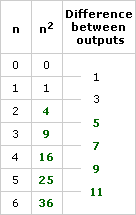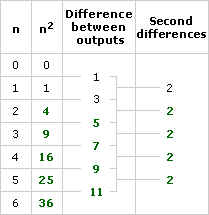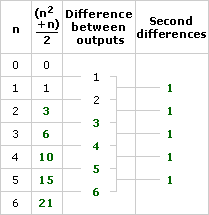# Nonlinear Functions Part D: Quadratic Functions (25 minutes)

## Session 7, Part D

### In This Part

• Summary

You know that for linear functions, the difference between successive outputs is a constant. For exponential functions, the ratio between successive outputs is a constant. Is there some similar pattern for quadratic functions? The next few problems will help you decide. Note 12

### Problem D1

As described at the end of Part C, quadratic functions involve squaring an input. The simplest quadratic function is simply output = (input)2. Here’s the start of a table for this function, with three columns: input, output, and the difference between successive outputs:

 nn2Difference between outputs 0 0 1 1 1 2 3 3 4 5 6

Fill in both the missing outputs and missing differences. Describe a pattern in the differences. Are the differences constant? Note 13

Tip: Remember, “constant” means the number remains the same: 5, 5, 5, … . A pattern may or may not be a constant pattern.

Problem D2

Add a new column to your table like the one shown below. In this column, put the “differences between differences,” called the second differences. What do you notice?

 nn2Difference between outputs Second differences 0 0 1 1 1 2 3 2 3 4 5 6

 Video Segment This video segment shows how to create a table of first differences and second differences in the equation y = x2. Watch this segment after you’ve completed Problem D2. If you get stuck on the problem, you can watch the video segment to help you. You can find this segment on the session video, approximately 15 minutes and 42 seconds after the Annenberg Media logo.

Problem D3

In Part C, you built a table for triangular numbers. One way to write the rule for triangular numbers is

output = (n2 + n) / 2.

Create a table for this rule for triangular numbers, and look for patterns in the first and second differences.

 n(n2 + n) 2Difference between outputs Second differences 0 0 1 1 1 2 3 2 3 4 5 6

A quadratic function is any function that can be written as…

output = A(input)2 + B(input) + C

A, B, and C can be any number. The only exception is that A cannot equal zero — if A were zero, there would be no need for the input to be squared!

Problem D4

Create your own quadratic function. Tabulate it and look at the differences and second differences. What seems to be true about quadratic functions?

 n Your quadratic functionDifference between outputs Second differences 0 1 2 3 4 5 6

Quadratic functions are studied in detail in physics and calculus, because a quadratic function describes anything falling under the force of gravity.

### Summary

In this session, we’ve seen some of the differences between linear functions, quadratic functions, and exponential functions and learned about their practical uses. The following Interactive Activity presents a graphical comparison between three functions that have similar equations but very different graphs:

y = 2x
y = x2
y = 2x

Problem D5

Which of these is an exponential function? Which is a quadratic function? Which is a linear function? For each, explain how you know.

### Notes

Note 12

In this last part of the session, we’ll look at differences between successive outputs of quadratic functions. Successive outputs do not produce constant differences (as with linear functions) or constant ratios (as with exponential functions), but the differences do have a pattern nonetheless. It turns out that for quadratic functions the differences are linear, and the second differences — the differences of differences — are constant.

Note 13

Groups: Work on Problems D1-D4 with a partner. Share results and describe the functions created for Problem D4. Out of this work, a conjecture should emerge that the second differences of quadratic functions are constant.

If there’s time, finish the session by looking for what the second difference tells you about the function. For a linear function, the difference between outputs is the same as the coefficient of x in the linear equation, and the same as the slope of the line.

Look at several different quadratic functions and the corresponding second differences. Notice that half of the second difference is the coefficient of the x2 term in the equation.

Looking back at the figurate numbers, for example, the square numbers had a formula y = x2. The coefficient of x2 is 1, and the second differences were constant 2s. The triangular numbers had a formula y = x2/2 + x/2. The coefficient of x2 is 1/2, and the second differences were constant 1s.

Look for the constant second differences for the pentagonal and hexagonal numbers, and use those to help find the more difficult formulas for these numbers.

Groups: Sharing results will allow you to collect information about quadratic functions. End the session by adding quadratic and exponential functions to the list of nonlinear functions started at the beginning of the session.

### Solutions

Problem D1

Here is the completed table:The differences are not constant, but they increase by 2 for each successive difference.

Problem D2

Here is the completed table:The difference between outputs forms a linear function, and the second differences are constant (in this case, they’re always equal to 2).

Problem D3

Here is the completed table:As before, the first differences form a linear function, and the second differences will always be constant.

Problem D4

You should find that with any quadratic function, the first differences will always form a linear function, and the second differences will always be constant.